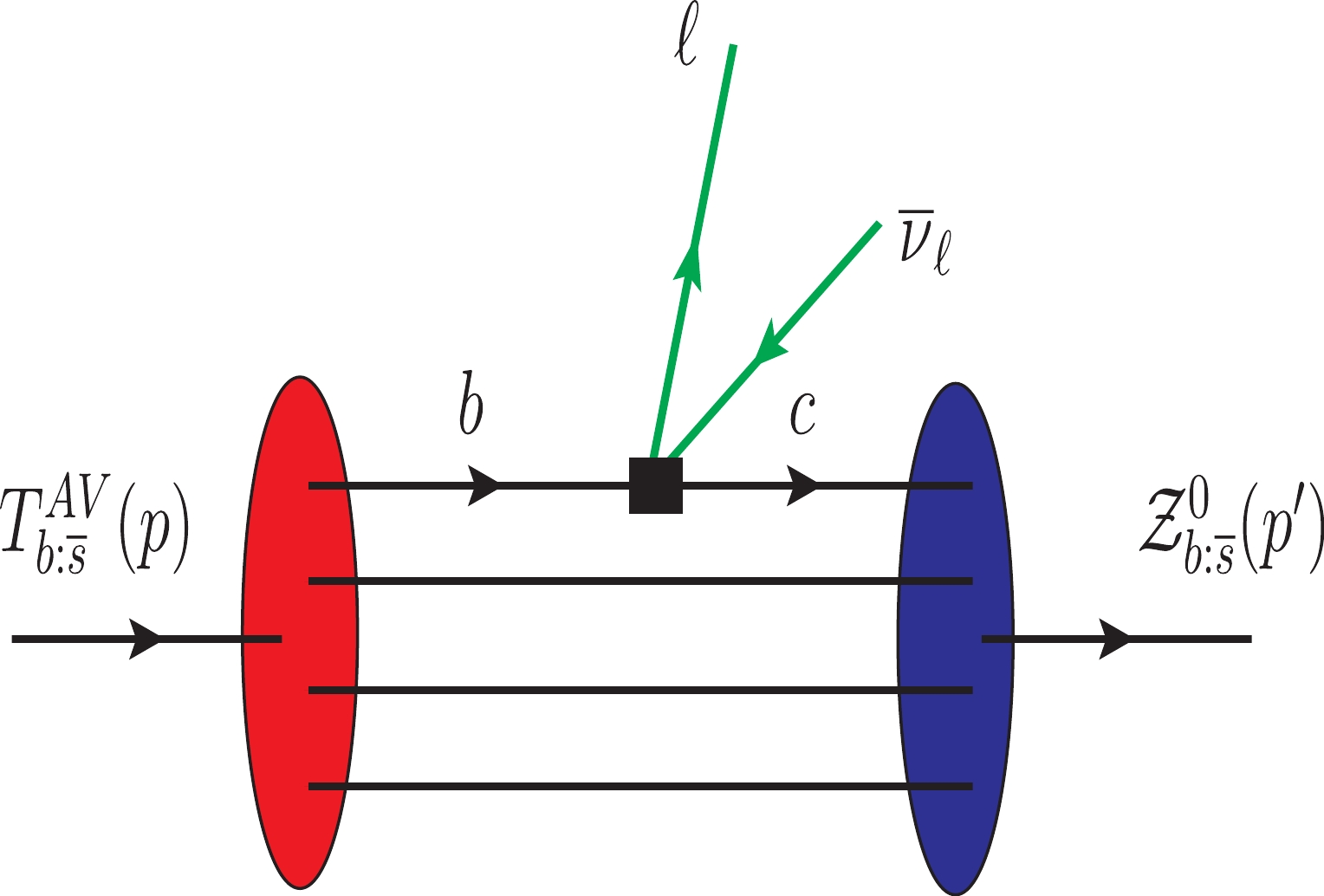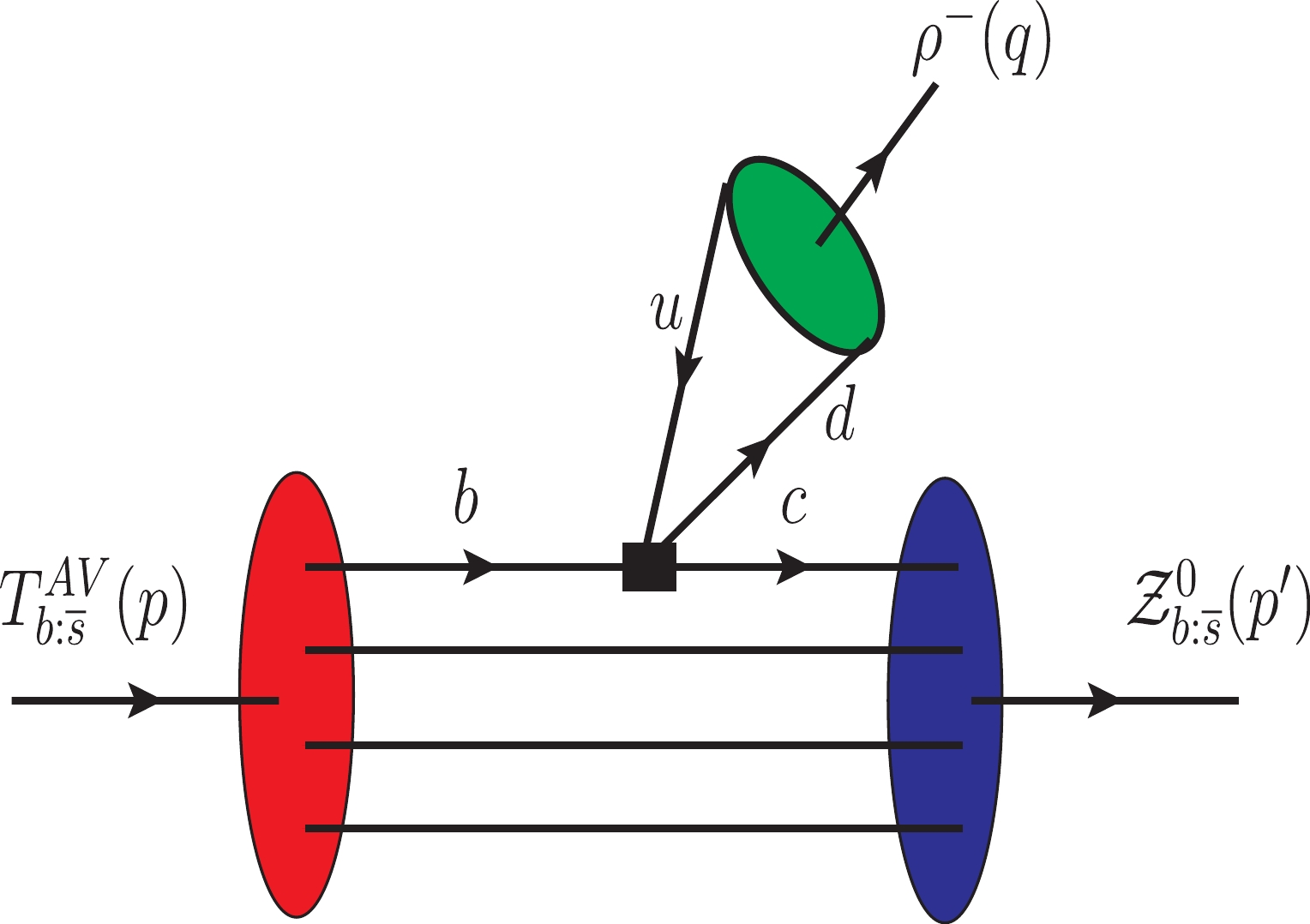# A family of double-beauty tetraquarks: Axial-vector state ${{T}^{{-}}_{{bb};\overline{{u}}\overline{{s}}}}}$• The spectroscopic parameters and decay channels of the axial-vector tetraquark $T_{bb;\overline{u}\overline{s}}^{-}$(in what follows, $T_{b:\overline{s}}^{\mathrm{AV}}$) are explored using the quantum chromodynamics (QCD) sum rule method. The mass and coupling of this state are calculated using two-point sum rules by taking into account various vacuum condensates, up to 10 dimensions. Our prediction for the mass of this state $m = (10215\pm 250)\; \mathrm{MeV}$confirms that it is stable with respect to strong and electromagnetic decays and can dissociate to conventional mesons only via weak transformations. We investigate the dominant semileptonic $T_{b:\overline{s}}^{\mathrm{AV}} \to {\cal{Z}}_{b:\overline{s}}^{0}l\overline{\nu}_l$and nonleptonic $T_{b:\overline{s}}^{\mathrm{AV}} \to {\cal{Z}}_{b:\overline{s}}^{0}M$decays of $T_{b:\overline{s}}^{\mathrm{AV}}$. In these processes, ${\cal{Z}}_{b:\overline{s}}^{0}$is a scalar tetraquark $[bc][\overline{u}\overline{s}]$built of a color-triplet diquark and an antidiquark, whereas M is one of the vector mesons $\rho ^{-}$, $K^{\ast}(892)$, $D^{\ast }(2010)^{-}$, and $D_{s}^{\ast -}$. To calculate the partial widths of these decays, we use the QCD three-point sum rule approach and evaluate the weak transition form factors $G_{i}$($i = 0,1,2,3$), which govern these processes. The full width $\Gamma _{\mathrm{full}} = (12.9\pm 2.1)\times 10^{-8}\; \mathrm{MeV}$and the mean lifetime $\tau = 5.1_{-0.71}^{+0.99}\; \mathrm{fs}$of the tetraquark $T_{b:\overline{s}}^{\mathrm{AV}}$are computed using the aforementioned weak decays. The obtained information about the parameters of $T_{b:\overline{s}}^{\mathrm{AV}}$and ${\cal{Z}}_{b:\overline{s}}^{0}$is useful for experimental investigations of these double-heavy exotic mesons.
••Get Citation
S. S. Agaev, K. Azizi, B. Barsbay and H. Sundu. A family of double-beauty tetraquarks: Axial-vector state ${{T}^{{-}}_{{bb};\overline{{u}}\overline{{s}}}}}$[J]. Chinese Physics C. doi: 10.1088/1674-1137/abc16d
S. S. Agaev, K. Azizi, B. Barsbay and H. Sundu. A family of double-beauty tetraquarks: Axial-vector state ${{T}^{{-}}_{{bb};\overline{{u}}\overline{{s}}}}}$[J]. Chinese Physics C.Milestone
Article Metric

Article Views(286)
Cited by(0)
Policy on re-use
To reuse of Open Access content published by CPC, for content published under the terms of the Creative Commons Attribution 3.0 license (“CC CY”), the users don’t need to request permission to copy, distribute and display the final published version of the article and to create derivative works, subject to appropriate attribution.
###### 通讯作者: 陈斌, bchen63@163.com
• 1.

沈阳化工大学材料科学与工程学院 沈阳 110142

Title:
Email:

## A family of double-beauty tetraquarks: Axial-vector state ${{T}^{{-}}_{{bb};\overline{{u}}\overline{{s}}}}}$• 1. Institute for Physical Problems, Baku State University, Az–1148 Baku, Azerbaijan
• 2. Department of Physics, University of Tehran, North Karegar Avenue, Tehran 14395-547, Iran
• 4. Department of Physics, Kocaeli University, 41380 Izmit, Turkey

Abstract: The spectroscopic parameters and decay channels of the axial-vector tetraquark $T_{bb;\overline{u}\overline{s}}^{-}$(in what follows, $T_{b:\overline{s}}^{\mathrm{AV}}$) are explored using the quantum chromodynamics (QCD) sum rule method. The mass and coupling of this state are calculated using two-point sum rules by taking into account various vacuum condensates, up to 10 dimensions. Our prediction for the mass of this state $m = (10215\pm 250)\; \mathrm{MeV}$confirms that it is stable with respect to strong and electromagnetic decays and can dissociate to conventional mesons only via weak transformations. We investigate the dominant semileptonic $T_{b:\overline{s}}^{\mathrm{AV}} \to {\cal{Z}}_{b:\overline{s}}^{0}l\overline{\nu}_l$and nonleptonic $T_{b:\overline{s}}^{\mathrm{AV}} \to {\cal{Z}}_{b:\overline{s}}^{0}M$decays of $T_{b:\overline{s}}^{\mathrm{AV}}$. In these processes, ${\cal{Z}}_{b:\overline{s}}^{0}$is a scalar tetraquark $[bc][\overline{u}\overline{s}]$built of a color-triplet diquark and an antidiquark, whereas M is one of the vector mesons $\rho ^{-}$, $K^{\ast}(892)$, $D^{\ast }(2010)^{-}$, and $D_{s}^{\ast -}$. To calculate the partial widths of these decays, we use the QCD three-point sum rule approach and evaluate the weak transition form factors $G_{i}$($i = 0,1,2,3$), which govern these processes. The full width $\Gamma _{\mathrm{full}} = (12.9\pm 2.1)\times 10^{-8}\; \mathrm{MeV}$and the mean lifetime $\tau = 5.1_{-0.71}^{+0.99}\; \mathrm{fs}$of the tetraquark $T_{b:\overline{s}}^{\mathrm{AV}}$are computed using the aforementioned weak decays. The obtained information about the parameters of $T_{b:\overline{s}}^{\mathrm{AV}}$and ${\cal{Z}}_{b:\overline{s}}^{0}$is useful for experimental investigations of these double-heavy exotic mesons.

### HTMLII.   SPECTROSCOPIC PARAMETERS OF THE AXIAL-VECTOR $T_{b:\overline{s}}^{\mathrm{AV}}$ AND SCALAR ${\cal{Z}}_{b:\overline{s}}^{0}$ TETRAQUARKSIII.   WEAK FORM FACTORS $G_{i}(p^{2})$ AND SEMILEPTONIC DECAYS $T_{b:\overline{s}}^{\mathrm{AV}}\rightarrow {\cal{Z}}_{b:\overline{s}}^{0}l\overline{\nu }_{l}$IV.   NONLEPTONIC DECAYS $T_{b:\overline{s}}^{\mathrm{AV}}\rightarrow {\cal{Z}}_{b:\overline{s}}^{0}M$V.   DISCUSSION AND CONCLUDING NOTESAPPENDIX A: THE PROPAGATORS $S_{q(Q)}(x)$ AND INVARIANT AMPLITUDE $\Pi (M^{2},s_{0})$
Reference (48)
PDF查看关注分享

Top

### 目录/DownLoad:  Full-Size Img  PowerPoint